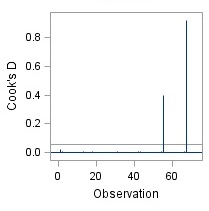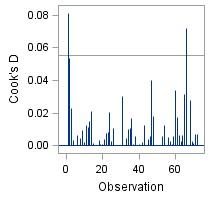## Pages

### Outlier Detection &Treatment - Part 2 - MultivariateWe have already covered basics about outliers and uni-variate approach for outlier detection in one of our previous articles.

In this article we would understand the multi-variate approach for outlier detection and then finally the outlier treatment methods.

I find the subject of multi-variate detection of outlier to be too difficult to make easy, in order to do so, a supporting article has been written covering practical application of uni-variate method and emphasizing on the need of multi-variate methods of outlier detection. Now we would take the topic forward, in conjunction of both the previous articles.

We strongly recommend you to go through following two articles sequentially, in order to harness best out of the this article.

All the questions we intended to cover in the article A:

1.  What are outliers?
2.  Why, at all, do we need to bother about outliers ?
3.  How to detect these outliers?
4.  How do we treat outliers?
5.  and last but not the least ... Is an outlier really an outlier ?

We have covered Question 1 and 2 completely and question 3 partially (uni-variate approach). It is time to understand the multi-variate approach.

### Multi-variate approach for outlier detection

1. X-Y Scatter plot :

X-Y Scatter plot is quite useful in analyzing a variable in conjunction with another variable.

Let's better understand the utility of this chart in outlier detection with an example. Suppose, we are analyzing Body Mass Index (BMI), which is calculated as Weight / Height of a person, and in order to do so, we first need to analyse height and weight variables.

We can plot weight and height together in X-Y scatter plot and create following charts:

In X-Y scatter plot, we can consider only two variables at a time. Hence it is a very time taking method, in case we need to analyze too many variables. We need something that can take care all the variables at once.

Presenting regression based methods ...

2. COOK's Distance :

Cook's distance is calculated during the process of Linear Regression. It is proxy of error at a particular observation in linear regression.
Suppose, If the x variables explain all other observation, but are unable to explain a particular point, the cook's distance at that point would be quite high and hence it indicates that the observation is outlier.

Consider the following data >>>  sales.sas7bdat

You can plot and see that there are two outliers in the data :  July 2011 and July 2012 months' observations.

While calculating the trend and seasonality these outliers are detected and treated by uni-variate methods, as explained in the article: Seasonality Index and Trend Variables.

Now, let's use the sales as such (with outliers) in regression :

Proc reg data = a.sales;
Model  Sales_with_Outlier = Distribution   Seasonality_Index   Trend  ;
Output out = result_sales p = predicted_sales  Cookd = cooks_distance ;Run;
Quit;

The option Cookd gives the observation wise calculated cook's distance in the output data. The cut-off of cook's distance is 4/N (where N = no. of observations). Any observation with a cook's distance >4/N is supposedly an outlier.

Also in the HTML output we get the plot of cook's distance with indication of presence of outliers :

Now looking at this plot, we can consider that there are two observations which are totally not being explained by the x variables and hence can be considered as outlier. We need to treat these.

The outliers can be of two types :
1. The reason for which is either known or can be identified with research,
2. Reason is totally unknown and can not be identified either.

The 2nd type of outliers should be either removed or can be treated with the mean of lag and lead ( explained in article :Seasonality Index and Trend Variables) or by any other methods. But for the 1st type of outliers, take a breath and think.

Think of the reasons of these observation being outlier, there must be some reason for sales being so high in these moths. Discuss with client!

Suppose, as a result of discussion with client, we get to know that in these months, their competitor's stock supply was short in market and because of which our client's brand got a chance to tap competitor's market share.
Now, while we know the reason, we can  use the dummy variable method for treating these 1st type of outliers.

We can create dummies for each observation that would capture the additional sales in the outlier months.

Data a.sales;
Set  a.sales;
compt_1 = 0;
if year = 2011 and month = 7 then compt_1 = 1;
compt_2 = 0;
if year = 2012 and month = 7 then compt_2 = 1;
Run;

Proc reg data =a.sales;
Model Sales_with_Outlier  =  Distribution Seasonality_Index Trend compt_1 compt_2 ;
Output out = result_sales p = predicted_sales  Cookd = cooks_distance ;
Run;Quit;

You can now see that the earlier  outlier's are no more outliers. New observation are now coming as outliers, however the degree of variation of cook's distance for outliers is not that extreme now. We can further investigate on these new outlier, or we can go ahead.Also, if you check the parameter estimates table, the coefficients of dummy variable compt_1 and compt_2  show the additional sales of our client's brand due to shortage in supply of competitor brand.

Want to treat all outliers at once ... even the mild ones ? Then go with :

3. Robust regression:

During OLS, if we find outliers we try to either treat these, remove these or balance their effect with a dummy (as seen above). However, the principle of robust regression is altogether different approach for dealing with outlier. The method considers different observation with different weightage, and provides outliers lesser weigthage; it is a mid-way between excluding the observation and including these as such in the model.

Looking at the weight statistics, we can identify the ouliers.

Proc RobustReg Data= a.Sales    method=m (wf=huber) ;
Model Sales_with_Outlier  =
Distribution
Seasonality_Index
Trend ;
Output out = rob_reg p = predicted weight=wgt;
Run;

Result :Click to enlarge

You can check in the resultant data, the outlier observations have been assigned quite small weights. Other observations have also been treated with lesser weightages if they are not fitting well with rest of the observations.

Try performing another model with Compt_1 and Compt_2 variables in robust regression. With these variables the extreme outlier observations won't be considered as outliers now and the special variation would be explained by these dummy variables.

This is quite handy method to find out outliers and perform regression on data infested with too many outliers.

Enjoy reading our other articles and stay tuned with ...

Kindly do provide your feedback in the 'Comments' Section and share as much as possible.

### Family of the article:

1. Outlier Detection &Treatment - A fresh perspective
2. Seasonality Index and Trend Variables
3. Outlier Detection &Treatment - Part 2 - Multivariate

#### 1 comment:

1.Do provide us your feedback, it would help us serve your better.